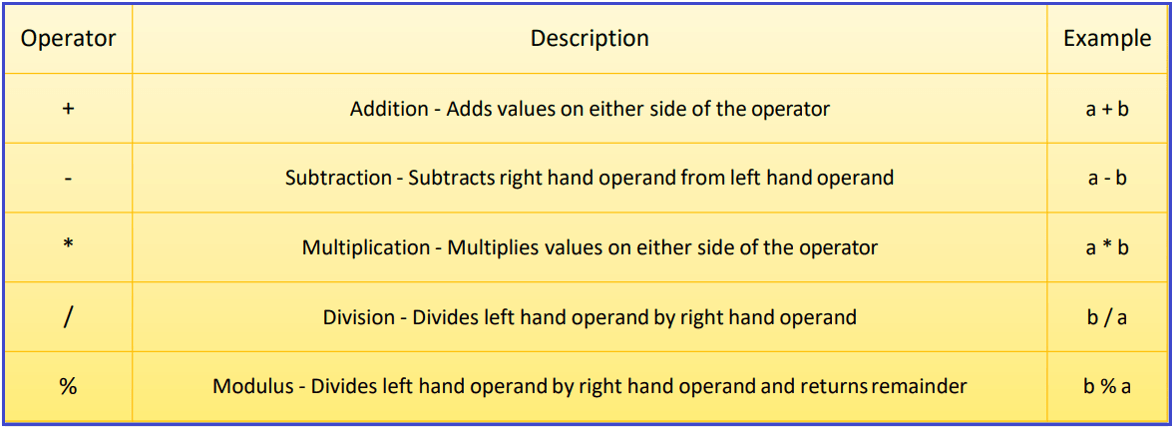# Arithmetic Operators in MySQL

## Arithmetic Operators in MySQL with Examples

In this article, I am going to discuss the Arithmetic Operators in MySQL with Examples. Please read our previous article where we discussed EXISTS Operator in MySQL with examples. At the end of this article, you will understand what are arithmetic operators and how to use different types of arithmetic operators in MySQL with examples.

##### What are Arithmetic Operators?

Arithmetic Operators are used for performing mathematical calculations such as Addition, Subtraction, Multiplication, Module, and Division represented by the expected +, -, *(star or asterisk), %, and / forward slash symbols respectively on the given operand values. That is, they are used to perform mathematical operations on two expressions of the same or different data types of numeric data.

The arithmetic operators can be directly used in the SQL statement or in combination with column values. The different arithmetic operators supported by MySQL are shown in the below image:##### Arithmetic Operators Example:
```SELECT 150 + 250; -- O/P = 400
SELECT 145 - 75; -- O/P = 70
SELECT 17 * 5; -- O/P = 85
SELECT 49 / 7; -- O/P = 7.0000
SELECT 21 % 5; -- O/P = 1```
##### How Arithmetic Operators are Evaluated in MySQL?

Similar to basic arithmetic calculations, the arithmetic operators in MySQL also have the same Operator Precedence. If the arithmetic expression contains more than one operator, then the multiplication and division operators are the highest priority and are evaluated first, and then the addition and minus (subtraction) operators are evaluated.

When two operators are having the same priority, then the expression is going to be evaluated from left to right in MySQL. The Parentheses in MySQL can also be used to force an operation to take priority over any other operators. Parentheses are also used to improve code readability.

##### Arithmetic Operators in MySQL Complex Queries:

We may not always retrieve the data as it is from the database tables. In some cases, we may need to display the data by doing some arithmetic operations. Let us understand how to use arithmetic operators with such complex SQL Queries in MySQL. We are going to use the following Employee table to understand this concept.Please use the below SQL Script to create the Company database and the Employee table with the required data.

```CREATE DATABASE Company;
USE Company;

CREATE TABLE Employee (
Id INT PRIMARY KEY,
Name VARCHAR(45) NOT NULL,
Department VARCHAR(45) NOT NULL,
Salary FLOAT NOT NULL,
Gender VARCHAR(45) NOT NULL,
Age INT NOT NULL,
City VARCHAR(45) NOT NULL
);

INSERT INTO Employee (Id, `Name`, Department, Salary, Gender, Age, City) VALUES (1001, 'John Doe', 'IT', 35000, 'Male', 25, 'London');
INSERT INTO Employee (Id, `Name`, Department, Salary, Gender, Age, City) VALUES (1002, 'Mary Smith', 'HR', 45000, 'Female', 27, 'London');
INSERT INTO Employee (Id, `Name`, Department, Salary, Gender, Age, City) VALUES (1003, 'James Brown', 'Finance', 50000, 'Male', 28, 'London');
INSERT INTO Employee (Id, `Name`, Department, Salary, Gender, Age, City) VALUES (1004, 'Mike Walker', 'Finance', 50000, 'Male', 28, 'London');
INSERT INTO Employee (Id, `Name`, Department, Salary, Gender, Age, City) VALUES (1005, 'Linda Jones', 'HR', 75000, 'Female', 26, 'London');
INSERT INTO Employee (Id, `Name`, Department, Salary, Gender, Age, City) VALUES (1006, 'Anurag Mohanty', 'IT', 35000, 'Male', 25, 'Mumbai');
INSERT INTO Employee (Id, `Name`, Department, Salary, Gender, Age, City) VALUES (1007, 'Priyanla Dewangan', 'HR', 45000, 'Female', 27, 'Mumbai');
INSERT INTO Employee (Id, `Name`, Department, Salary, Gender, Age, City) VALUES (1008, 'Sambit Mohanty', 'IT', 50000, 'Male', 28, 'Mumbai');
INSERT INTO Employee (Id, `Name`, Department, Salary, Gender, Age, City) VALUES (1009, 'Pranaya Kumar', 'IT', 50000, 'Male', 28, 'Mumbai');
INSERT INTO Employee (Id, `Name`, Department, Salary, Gender, Age, City) VALUES (1010, 'Hina Sharma', 'HR', 75000, 'Female', 26, 'Mumbai');
```
##### Example: Arithmetic Operators

Our requirement is to calculate the salaries of each employee by adding 10% as a bonus. The following SQL Query will calculate the employee’s salary after the addition of 10% and show it in the Bonus along with the Id, Name, Salary column.

SELECT ID, Name, Salary, Salary * 1.1 AS Bonus FROM Employee;

Once you execute the above query, you will get the following result set.It is also possible to perform multiple mathematical calculations as shown in the below example.

SELECT ID, Name, Salary, Salary * 0.11 / 2.54 + 27.36 AS Bonus FROM Employee;

Once you execute the above query, you will get the following result set.If you want to provide the order precedence of the arithmetic operators then simply use round brackets as shown in the below example.

SELECT ID, Name, Salary, Salary * 0.11 / (2.54 + 27.36) AS Bonus FROM Employee;

Once you execute the above query, you will get the following result set.Note: MySQL Ignores blank spaces before and after the arithmetic operators. Along with, in MySQL, we can also use arithmetic operators on multiple columns.

In the next article, I am going to discuss Concatenation and Temporal Operators in MySQL with Examples. Here, in this article, I try to explain Arithmetic Operators in MySQL with Examples. I hope you enjoy this article.